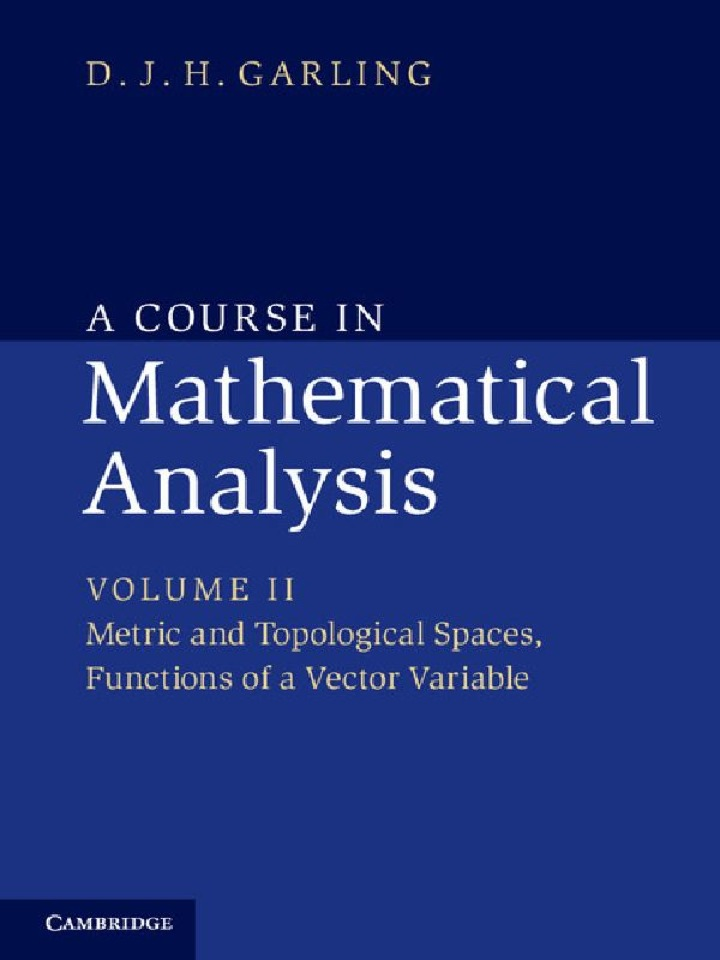# A Course in Mathematical Analysis: Volume 2, Metric and Topological Spaces, Functions of a Vector Variable

## A Course in Mathematical Analysis: Volume 2, Metric and Topological Spaces, Functions of a Vector Variable

By D. J. H. Garling

### Rent or Buy eTextbook

Expires on Apr 18th, 2022
\$43

Publisher List Price: \$0.00

The three volumes of A Course in Mathematical Analysis provide a full and detailed account of all those elements of real and complex analysis that an undergraduate mathematics student can expect to encounter in their first two or three years of study. Containing hundreds of exercises, examples and applications, these books will become an invaluable resource for both students and teachers. Volume 1 focuses on the analysis of real-valued functions of a real variable. This second volume goes on to consider metric and topological spaces. Topics such as completeness, compactness and connectedness are developed, with emphasis on their applications to analysis. This leads to the theory of functions of several variables. Differential manifolds in Euclidean space are introduced in a final chapter, which includes an account of Lagrange multipliers and a detailed proof of the divergence theorem. Volume 3 covers complex analysis and the theory of measure and integration.

Subject: Mathematics & Statistics -> Mathematics -> Mathematics General1st edition
Publisher: Cambridge University Press 1/23/14
Imprint: Cambridge University Press
Language: English

ISBN 10: 1107357926
ISBN 13: 9781107357921
Print ISBN: 9781107032033

Live Chats